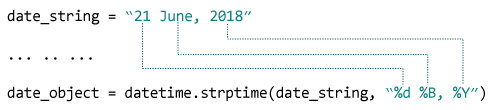# Python strptime()

In this article, you will learn to create a datetime object from a string (with the help of examples).

The `strptime()` method creates a datetime object from the given string.

Note: You cannot create `datetime` object from every string. The string needs to be in a certain format.

## Example 1: string to datetime object

``````
from datetime import datetime

date_string = "21 June, 2018"

print("date_string =", date_string)
print("type of date_string =", type(date_string))

date_object = datetime.strptime(date_string, "%d %B, %Y")

print("date_object =", date_object)
print("type of date_object =", type(date_object))
``````

When you run the program, the output will be:

```
date_string = 21 June, 2018
type of date_string = <class 'str'>
date_object = 2018-06-21 00:00:00
type of date_object = <class 'datetime.datetime'>
```

### How strptime() works?

The `strptime()` class method takes two arguments:

• string (that be converted to datetime)
• format code

Based on the string and format code used, the method returns its equivalent `datetime` object.

In the above example:Here,

• `%d` - Represents the day of the month. Example: 01, 02, ..., 31
• `%B` - Month's name in full. Example: January, February etc.
• `%Y` - Year in four digits. Example: 2018, 2019 etc.

## Example 2: string to datetime object

``````
from datetime import datetime

dt_string = "12/11/2018 09:15:32"

# Considering date is in dd/mm/yyyy format
dt_object1 = datetime.strptime(dt_string, "%d/%m/%Y %H:%M:%S")
print("dt_object1 =", dt_object1)

# Considering date is in mm/dd/yyyy format
dt_object2 = datetime.strptime(dt_string, "%m/%d/%Y %H:%M:%S")
print("dt_object2 =", dt_object2)
``````

When you run the program, the output will be:

```dt_object1 = 2018-11-12 09:15:32
dt_object2 = 2018-12-11 09:15:32
```

## Format Code List

The table below shows all the format codes that you can use.

 Directive Meaning Example `%a` Abbreviated weekday name. Sun, Mon, ... `%A` Full weekday name. Sunday, Monday, ... `%w` Weekday as a decimal number. 0, 1, ..., 6 `%d` Day of the month as a zero-padded decimal. 01, 02, ..., 31 `%-d` Day of the month as a decimal number. 1, 2, ..., 30 `%b` Abbreviated month name. Jan, Feb, ..., Dec `%B` Full month name. January, February, ... `%m` Month as a zero-padded decimal number. 01, 02, ..., 12 `%-m` Month as a decimal number. 1, 2, ..., 12 `%y` Year without century as a zero-padded decimal number. 00, 01, ..., 99 `%-y` Year without century as a decimal number. 0, 1, ..., 99 `%Y` Year with century as a decimal number. 2013, 2019 etc. `%H` Hour (24-hour clock) as a zero-padded decimal number. 00, 01, ..., 23 `%-H` Hour (24-hour clock) as a decimal number. 0, 1, ..., 23 `%I` Hour (12-hour clock) as a zero-padded decimal number. 01, 02, ..., 12 `%-I` Hour (12-hour clock) as a decimal number. 1, 2, ... 12 `%p` Locale’s AM or PM. AM, PM `%M` Minute as a zero-padded decimal number. 00, 01, ..., 59 `%-M` Minute as a decimal number. 0, 1, ..., 59 `%S` Second as a zero-padded decimal number. 00, 01, ..., 59 `%-S` Second as a decimal number. 0, 1, ..., 59 `%f` Microsecond as a decimal number, zero-padded on the left. 000000 - 999999 `%z` UTC offset in the form +HHMM or -HHMM. `%Z` Time zone name. `%j` Day of the year as a zero-padded decimal number. 001, 002, ..., 366 `%-j` Day of the year as a decimal number. 1, 2, ..., 366 `%U` Week number of the year (Sunday as the first day of the week). All days in a new year preceding the first Sunday are considered to be in week 0. 00, 01, ..., 53 `%W` Week number of the year (Monday as the first day of the week). All days in a new year preceding the first Monday are considered to be in week 0. 00, 01, ..., 53 `%c` Locale’s appropriate date and time representation. Mon Sep 30 07:06:05 2013 `%x` Locale’s appropriate date representation. 09/30/13 `%X` Locale’s appropriate time representation. 07:06:05 `%%` A literal '%' character. %

### ValueError in strptime()

If the string (first argument) and the format code (second argument) passed to the `strptime()` doesn't match, you will get `ValueError`. For example:

``````
from datetime import datetime

date_string = "12/11/2018"
date_object = datetime.strptime(date_string, "%d %m %Y")

print("date_object =", date_object)
``````

If you run this program, you will get an error.

```ValueError: time data '12/11/2018' does not match format '%d %m %Y'
```